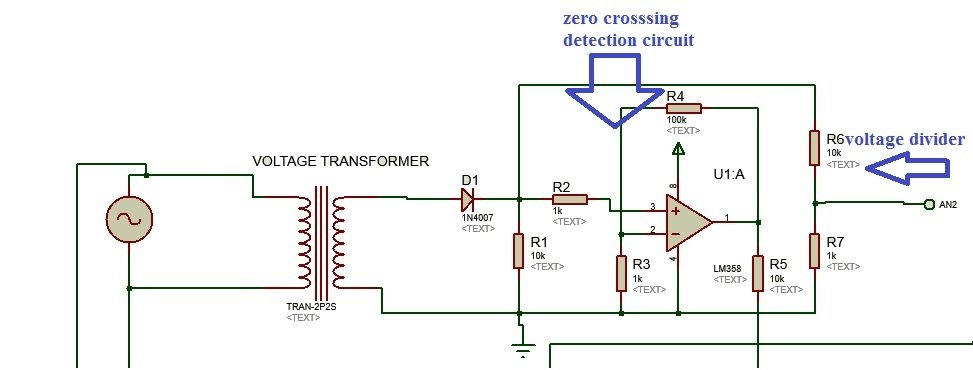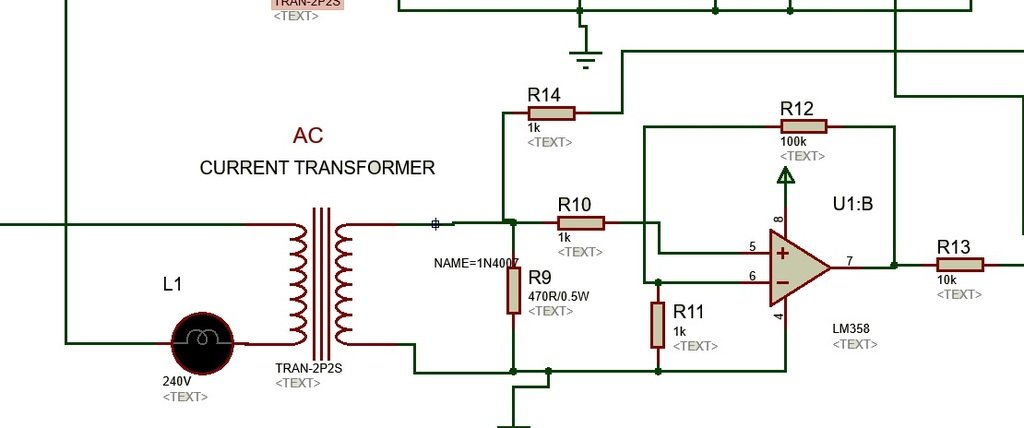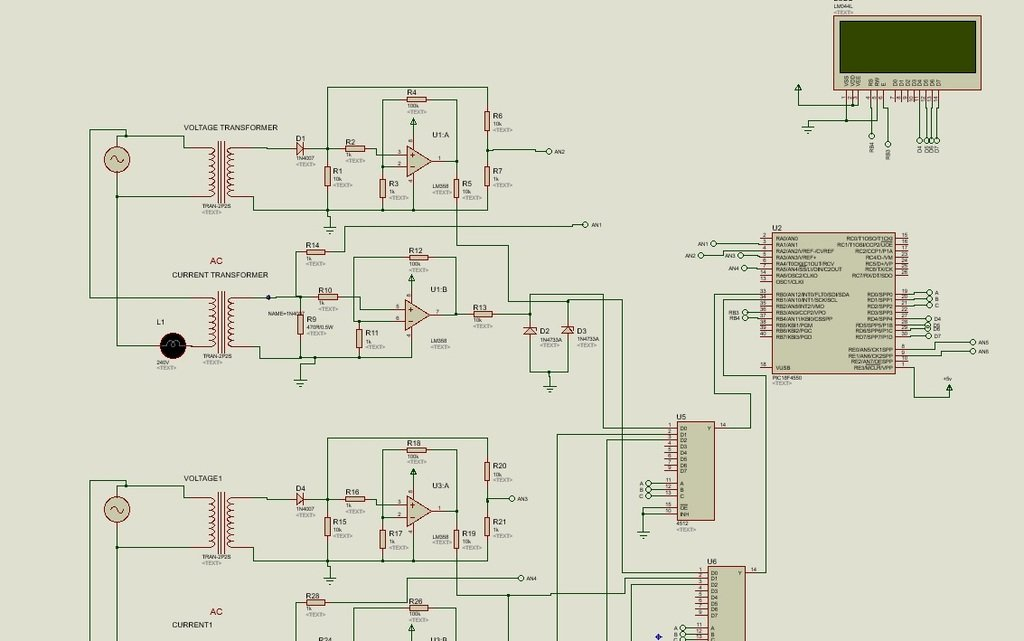# Three phase ac power measurement using pic microcontroller

Three phase ac power measurement: three phase watt meter using pic microcontroller is designed to measure three phase ac power of three phase transformer and three phase generator. It can be used to measure either three phase ac power of transformer or three phase generator. As you know AC power or wattage of any three phase ac equipment is difficult to measure with the help of microcontroller due to non linearity and out of phase behavior of voltage and current wave forms.  I will explain it in more detail in later part of this article.  three phase watt meters has many applications in industry. Three phase watt meters are used to measure power consumed by three phase induction machines and they are also used in power system as energy meter to measure power consumed by the user.So Now lets get start with basic components used in this project.

### Component of three phase watt meter

Following are the main components used in three phase ac power measurement system using pic microcontroller.

PIC18F4550 pic microcontroller: It is the main part of this project. It is used to measure three phase ac power. It measures current, voltage and phase angle between voltage and current.  Power is calculated using product of voltage, current and power factor. It also displays value of current, voltage, ac power and frequency of each phase on LCD. you can read a article on LCD interfacing with pic16f877a microcontroller.

AC voltage measurement circuit : AC voltage measurement circuit is designed using step down transformer, full bridge rectifier and voltage divider as shown below. It also has a zero crossing detection circuit using operational amplifier. Microcontroller can not measure voltage more than 5 volt. So wee need to use a step down transformer which converts 220 volt AC into 12 volt AC. But this 12 Volt AC also has negative cycle, so we used have bridge rectifier circuit to remove negative cycle.

Because microcontroller can not read negative voltage either. After that we use a voltage divider which further step down the 12 volt ac to below 5 volt which is in acceptable range of pic microcontroller analog to digital converter. Built in analog channel pic microcontroller measured peak value of positive half cycle and this peak voltage is converter back into rms voltage using formula of peak voltage to RMS voltage conversion.Current measurement circuit : Current measurement circuit also works on same principle as voltage measurement circuit except current transformer is used instead of voltage transformer as shown in circuit diagram. Current transformer is used to step down high current into low current. 100A/10A step down current transformer can be used. After current transformer, a resistor is used, voltage drop across this resistor is used to measure current with the help of built in adc of pic microcontroller. Zero crossing detector is also used with current measurement circuit.  I will explain later the need of zero crossing detectorZero crossing detector circuit : Zero crossing detector circuit in three phase watt meter converts zero crossing signals to square waves. Time difference in both square waves are use to measure time delay between voltage and current wave forms. This time delay is ultimately used to measure power factor. Timers of pic microcontroller are used to measure time difference. Measured power factor is used to calculate power of each phase.

### Circuit diagram of three phase watt meter using pic microcontroller

Circuit diagram of three phase watt meter using pic microcontroller is given. I have explained the necessary components of this circuit diagram above.  Three voltage measurement circuits are used and three current measurement circuit are used.  LCD is used to display values of current, voltage, power and frequency of each phase.### 6 thoughts on “Three phase ac power measurement using pic microcontroller”

1. sir is ka program b write krain please.